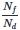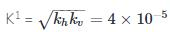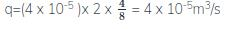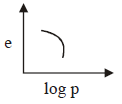Courses

# Test: Soil Mechanics - 1

## 25 Questions MCQ Test Civil Engineering SSC JE (Technical) | Test: Soil Mechanics - 1

Description
This mock test of Test: Soil Mechanics - 1 for Civil Engineering (CE) helps you for every Civil Engineering (CE) entrance exam. This contains 25 Multiple Choice Questions for Civil Engineering (CE) Test: Soil Mechanics - 1 (mcq) to study with solutions a complete question bank. The solved questions answers in this Test: Soil Mechanics - 1 quiz give you a good mix of easy questions and tough questions. Civil Engineering (CE) students definitely take this Test: Soil Mechanics - 1 exercise for a better result in the exam. You can find other Test: Soil Mechanics - 1 extra questions, long questions & short questions for Civil Engineering (CE) on EduRev as well by searching above.
QUESTION: 1

Solution:
QUESTION: 2

Solution:
QUESTION: 3

### Clay can be classified as

Solution:
QUESTION: 4

As per ISS the specific gravity of soil is determined at

Solution:
QUESTION: 5

The degree of saturation in soils can be defined as the ratio of –

Solution:
QUESTION: 6

When the pores of a soil are full of water then the soil sill be called –

Solution:
QUESTION: 7

A dry soil has a mass specific gravity of 1.35. It the specific gravity of solids is 2.7. then the voids ratio will be –

Solution:
QUESTION: 8

The specific gravity of sandy soil is –

Solution:
QUESTION: 9

The natural water content of the soil sample was found to be 40%, specific gravity is 2.7 and void ratio 1.2 then the degree of saturation of the soil will be –

Solution:
QUESTION: 10

A functional equation of specific gravity (G) water content (w), void ratio (e) and degree of
saturation (sr) is :-

Solution:
QUESTION: 11

The soil having unitormity coefficient less than 4 is called

Solution:
QUESTION: 12

The effective size of soil is

Solution:
QUESTION: 13

The uniformity co-efficient of soil is defined as the ratio of -

Solution:
QUESTION: 14

A flow net for seepage under a sheet pile wall has nf = 4 nd = 8 and the permeabilities of the soil in the horizontal direction are kh = 8× 10–5 m/sec and kv = = 2 × 10-5 m/sec. If the head loss through the soil is 2m, the quantity of seepage per meter length of the wall will be -

Solution:

For a sheet pile the quantity of seepage per unit length of wall is given by q=k1.HQUESTION: 15

The plastic limit exists in case of

Solution:
QUESTION: 16

The percentage of porosity of loose sand is in the range -

Solution:
QUESTION: 17

The shear strength of a soil in the liquid limit state is –

Solution:
QUESTION: 18

The plastic index is

Solution:
QUESTION: 19

When the plastic limit of a soil is greater than the liquid limit, then the plasticity index is –

Solution:
QUESTION: 20

The law that states that laminar flow in a saturated soil, the velocity is directly proportional to hydraulic gradient is called –

Solution:
QUESTION: 21

The dimensions of K are –

Solution:
QUESTION: 22

K is proportional to 'e' as

Solution:
QUESTION: 23

The e-log p curve shown in the figure is representative of –Solution:
QUESTION: 24

Which of the following is not an assumption in the terzaghic's theory of one diamensional consolidation

Solution:
QUESTION: 25

A clay sample has a void ratio of 0.50 in dry state and specific gravity of solids = 2.70 its shrinkage limit will be –

Solution: